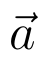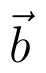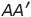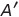Select Page

# Maths Vector Algebra CBSE 12 Science Solutions for MCQ in English

Maths Vector Algebra CBSE 12 Science Solutions for MCQ in English to enable students to get Solutions in a narrative video format for the specific question.

Expert Teacher provides Maths Vector Algebra CBSE 12 Science Solutions for MCQ through Video Solutions in English language. This video solution will be useful for students to understand how to write an answer in exam in order to score more marks. This teacher uses a narrative style for a question from Vector Algebra not only to explain the proper method of answering question, but deriving right answer too.

Please find the question below and view the Solution in a narrative video format.

Question:

Solution Video in English:

You can select video Solutions from other languages also. Please check Solutions in ( Hindi )

## Similar Questions from CBSE, 12th Science, Maths, Vector Algebra

Question 1 : Find a vector in the direction ofthat has magnitude 7 units.   (View Answer Video)

Question 2 : Find the unit vector in the direction of the vector(View Answer Video)

Question 3 : Find the vector quantities :
(i)     Work                     (ii)      Force

Question 4 :  Ifanddenote the position vectors of points A and B respectively and C is a point on AB such that AC = 2 CB, then write the position vector of C.  (View Answer Video)

Question 5 : Find the vector quantities from the following:
(i) Time period          (ii) Distance          (iii) Force
(iv) Velocity               (v) Work done

### Continuity and Differentiability

Question 1 : Findfor the function. (View Answer Video)

Question 2 : Differentiate the functionwith respect to x. (View Answer Video)

Question 3 : Findfor the function. (View Answer Video)

Question 4 : Differentiate the function w.r.t.x. (View Answer Video)

Question 5 : Differentiate the function w.r.t.x. (View Answer Video)

### Inverse Trigonometric Functions

Question 1 :is equal to :

Question 2 : Solve for(View Answer Video)

Question 3 : Solve the equation :(View Answer Video)

Question 4 : Solve for(View Answer Video)

Question 5 : Solve for(View Answer Video)

### Matrices

Question 1 : Find the value of x, if. (View Answer Video)

Question 2 : Find the value of c from the equation:(View Answer Video)

Question 3 : If,find the value of x. (View Answer Video)

Question 4 : If, write, whereis the transpose of A? (View Answer Video)

Question 5 : Find the value of X, ifand. (View Answer Video)